## How Much Force Applied to a Rig Tong To Get The Right Torque at The ConnectionOne application of the rig tong is to use to make up connection. The question is asked about how to get the right torque value to the connection if you use the rig tong because you will not see the torque value on the gauge. This article will describe how to determine the correct force applied to get the correct torque value when you use the rig tong to make up the connection.This formula below is used to determine force at required make up torque value.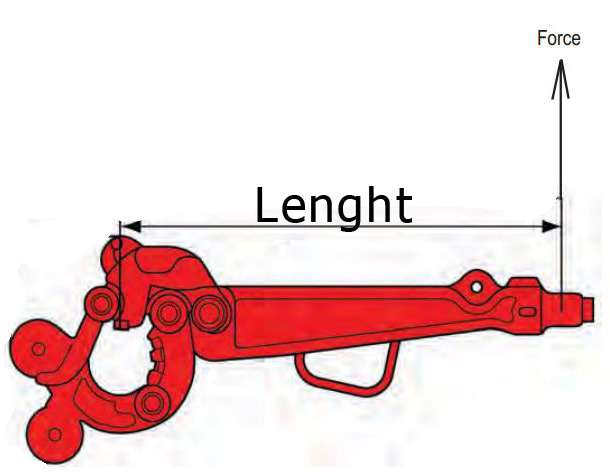# Oilfield Unit

## Force = Torque ÷ Length of the tong

Where;

Torque in ft-lb

Force in lb

Length of the tong in ft

Force is perpendicular to the tong length.

The illustration above demonstrates the direction of force.

# Metric Unit

## Force = Torque ÷ Length of the tong

Where;

Torque in N-m

Force in

Length of the tong in m

Force is perpendicular to the tong length.

Example:

Connection 4-1/2” IF – required make up torque = 30,700 ft-lb.

Tong length is 4 ft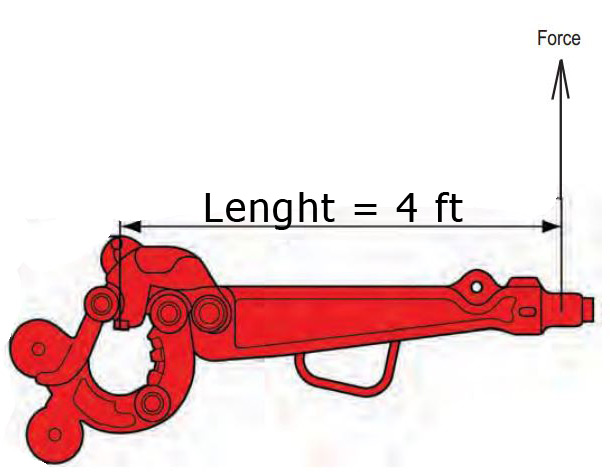How much pulling force do you require to achieve the required make up torque?

Force = Torque ÷ Length of the tong

Force = 30,700 ÷ 4.0

Force = 7,675 lb

Answer: 7,675 lb pulling force is applied to 4-ft tong in order to get the torque at connection at 30,700 ft-lb.Ref books:

Lapeyrouse, N.J., 2002. Formulas and calculations for drilling, production and workover, Boston: Gulf Professional publishing.

Bourgoyne, A.J.T., Chenevert , M.E. & Millheim, K.K., 1986. SPE Textbook Series, Volume 2: Applied Drilling Engineering, Society of Petroleum Engineers.

Mitchell, R.F., Miska, S. & Aadny, B.S., 2011. Fundamentals of drilling engineering, Richardson, TX: Society of Petroleum Engineers.

## Coring Cost Per Footage Drilled

Coring is a special process to recover wellbore rock in the well.Figure 1 – Core from the well. Credit – Wikipedia

This article will demonstrate how to calculate coring cost per footage recovered.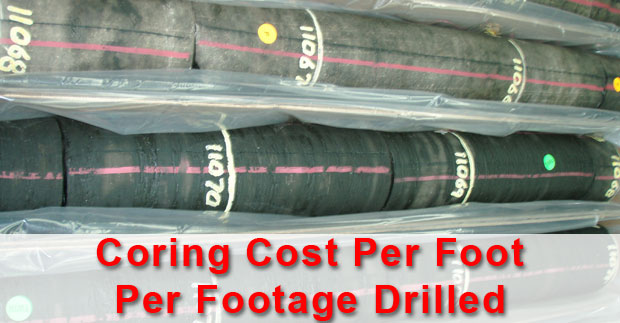Coring cost per footage recovered is expressed below;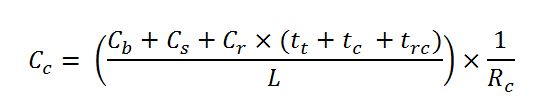## Volume of Cutting Generated While Drilling

While drilling, cuttings are generated every footage drilled and this topic will demonstrate how to determine volume of cutting entering into the wellbore.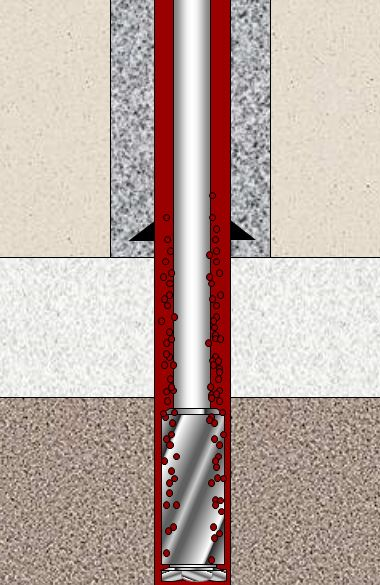Figure 1 – Cutting Generated While Drilling

The following formula is used to calculate cutting volume generated while drilling;Where;

Vc is volume of cutting in bbl/hr.

Ø is formation porosity (%).

D is wellbore diameter in inch.

ROP is rate of penetration in feet per hour.

Vc can be presented in several unit as follows;

## Buoyancy Factor with Two Different Fluid Weights in The WellBuoyancy Factor is the factor that is used to compensate loss of weight due to immersion in drilling fluid and you can find more information from this article > buoyancy factor calculation .  In that article, it demonstrates the buoyancy formula only for one fluid in the wellbore. However, this time, we will have the details about buoyancy factor when inside and outside fluid are different.

Buoyancy factor with different fluid inside and outside of tubular is listed below;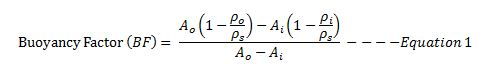Where;

Ao is an external area of the component.

Ai is an internal area of the component.

ρo is fluid density in the annulus at the component depth in the wellbore.

ρi is fluid density in the component depth in the wellbore.

ρs is steel weight density. Steel density is 65.4 ppg.

If you can the same mud weight inside and outside, the equation 1 will be like this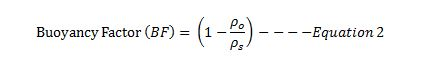Let’s take a look at the following example to get more understanding.

Example

13-3/8” casing shoe was at 2,500’MD/2,000’TVD

9-5/8” casing was run to 6,800’MD/6,000 TVD.

9-5/8” casing weight is 40 ppf and casing ID is 8.835 inch.

Current mud weight is 9.5 ppg oil based mud.

The well bore diagram is show below (Figure 1).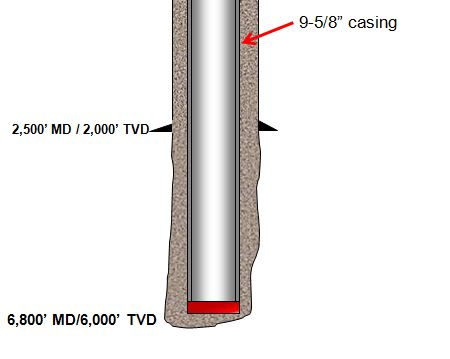Figure 1 – Wellbore Diagram

The well is planned to cement from shoe to surface and the planned cement weight is 14.0 ppg. The displacement fluid is drilling mud currently used.

• Air weight of casing string
• Buoyed weight of casing in drilling mud
• Buoyed weight of casing when cement is inside casing and drilling mud is outside casing
• Buoyed weight of casing when cement is outside casing and drilling mud is inside casing

Air weight of casing string

Air weight of casing string, lb = length of casing, ft × casing weight, lb/ft

Air weight of casing string, lb = 6,800 × 40 = 272,000 lb

Buoyed weight of casing in drilling mudFigure 2 – Buoyed Weight When Submersed In Drilling Mud

Buoyed weight = Buoyancy Factor (BF) × Air Weight of Casing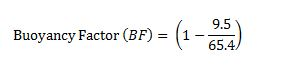Buoyancy Factor (BF) = 0.855

Buoyed weight = 0.855 × 272,000 = 232,489 lb

Buoyed weight of casing when cement is inside casing and drilling mud is outside casing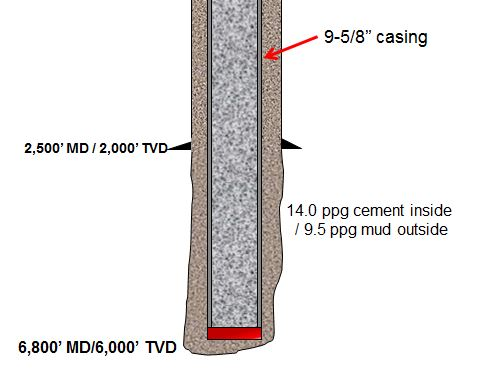Figure 3 – Buoyed weight of casing when cement is inside casing and drilling mud is outside casing

We will apply the Equation-1 for this case.

Ao is an external area of the component.

Ao = π × (Outside Diameter of casing)2 ÷ 4

Ao = π × (9.625)2 ÷ 4 = 72.76 square inch

Ai is an internal area of the component.

Ai = π × (Inside Diameter of casing)2 ÷ 4

Ai = π × (8.835)2 ÷ 4 = 61.31 square inch

ρo = 9.5 ppf (mud in the annulus)

ρi = 14.0 ppg (cement inside casing)

ρs = 65.4 ppg.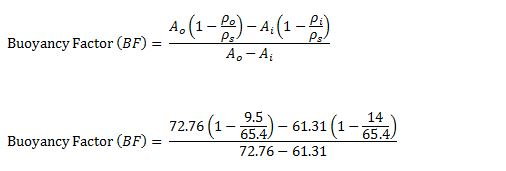Buoyancy Factor (BF) = 1.22

Buoyed weight = 1.22 × 272,000 = 331,840 lb

Buoyed weight of casing when cement is outside casing and drilling mud is inside casing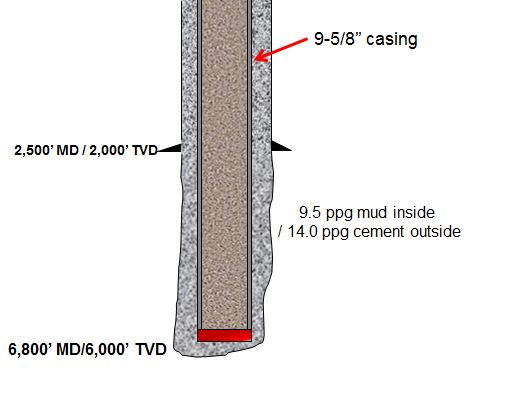Figure 4 – Buoyed weight of casing when cement is outside casing and drilling mud is inside casing

We will apply Equation-1 for this case as well.

All the calculation parameters are the same.

Ao = π × (9.625)2 ÷ 4 = 72.76 square inch

Ai = π × (8.835)2 ÷ 4 = 61.31 square inch

ρo = 9.5 ppg (mud in the annulus)

ρi = 14.0 ppg (cement inside casing)

ρs = 65.4 ppg.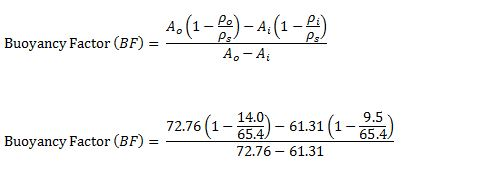Buoyancy Factor (BF) = 0.42

Buoyed weight = 0.42 × 272,000 = 114,240 lb

Conclusion: At different stage of the well, you may have different buoyed weight depending on density of fluid inside and outside of the component and it is not always that buoyed weight is less than air weight.

Reference book =>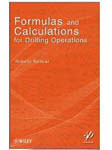Formulas and Calculations for Drilling Operations

## Maximum ROP Before Fracture Formation

In this topic, we will apply the effective mud density formula to determine maximum ROP before fracturing formation.Figure 1 – Max ROP before Fracturing Formation

These two equations that will be used to determine the maximum ROP are listed below;

Effective mud density due to cuttings in the hole can be determined by the empirical equation below;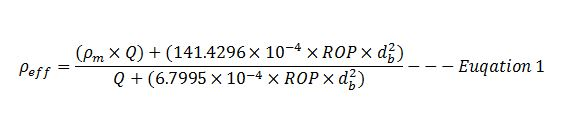Where;

ρeff is effective mud density in ppg.

ρm is mud density in ppg.

Q is flow rate in gpm.

ROP is rate of penetration in fph.

db is wellbore diameter or bit diameter in inch.